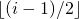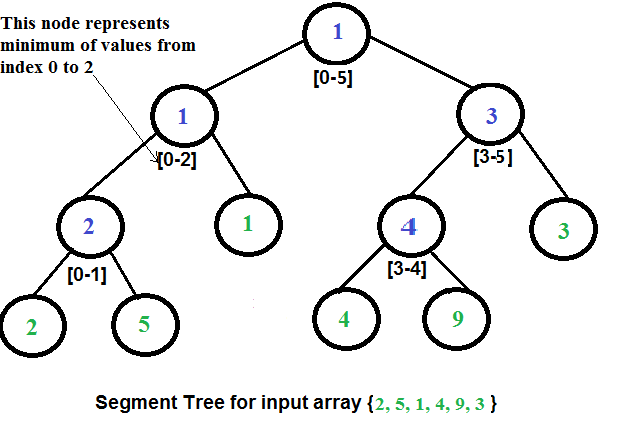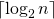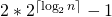Skip to content
Related Articles
Segment Tree | Set 2 (Range Minimum Query)
• Difficulty Level : Hard
• Last Updated : 22 Nov, 2019

We have introduced segment tree with a simple example in the previous post. In this post, Range Minimum Query problem is discussed as another example where Segment Tree can be used. Following is problem statement.

We have an array arr[0 . . . n-1]. We should be able to efficiently find the minimum value from index qs (query start) to qe (query end) where 0 <= qs <= qe <= n-1.

## Recommended: Please solve it on “PRACTICE” first, before moving on to the solution.

A simple solution is to run a loop from qs to qe and find minimum element in given range. This solution takes O(n) time in worst case.

Another solution is to create a 2D array where an entry [i, j] stores the minimum value in range arr[i..j]. Minimum of a given range can now be calculated in O(1) time, but preprocessing takes O(n^2) time. Also, this approach needs O(n^2) extra space which may become huge for large input arrays.

Segment tree can be used to do preprocessing and query in moderate time. With segment tree, preprocessing time is O(n) and time to for range minimum query is O(Logn). The extra space required is O(n) to store the segment tree.

Representation of Segment trees
1. Leaf Nodes are the elements of the input array.
2. Each internal node represents minimum of all leaves under it.

An array representation of tree is used to represent Segment Trees. For each node at index i, the left child is at index 2*i+1, right child at 2*i+2 and the parent is at.Construction of Segment Tree from given array
We start with a segment arr[0 . . . n-1]. and every time we divide the current segment into two halves(if it has not yet become a segment of length 1), and then call the same procedure on both halves, and for each such segment, we store the minimum value in a segment tree node.
All levels of the constructed segment tree will be completely filled except the last level. Also, the tree will be a Full Binary Tree because we always divide segments in two halves at every level. Since the constructed tree is always full binary tree with n leaves, there will be n-1 internal nodes. So total number of nodes will be 2*n – 1.
Height of the segment tree will be. Since the tree is represented using array and relation between parent and child indexes must be maintained, size of memory allocated for segment tree will be.

Query for minimum value of given range
Once the tree is constructed, how to do range minimum query using the constructed segment tree. Following is algorithm to get the minimum.

```// qs --> query start index, qe --> query end index
int RMQ(node, qs, qe)
{
if range of node is within qs and qe
return value in node
else if range of node is completely outside qs and qe
return INFINITE
else
return min( RMQ(node's left child, qs, qe), RMQ(node's right child, qs, qe) )
}```

Implementation:

## C++

 `// C++ program for range minimum``// query using segment tree ``#include ``using` `namespace` `std;`` ` `// A utility function to get minimum of two numbers ``int` `minVal(``int` `x, ``int` `y) { ``return` `(x < y)? x: y; } `` ` `// A utility function to get the ``// middle index from corner indexes. ``int` `getMid(``int` `s, ``int` `e) { ``return` `s + (e -s)/2; } `` ` `/* A recursive function to get the``minimum value in a given range ``of array indexes. The following ``are parameters for this function. `` ` `    ``st --> Pointer to segment tree ``    ``index --> Index of current node in the ``           ``segment tree. Initially 0 is ``           ``passed as root is always at index 0 ``    ``ss & se --> Starting and ending indexes ``                ``of the segment represented ``                ``by current node, i.e., st[index] ``    ``qs & qe --> Starting and ending indexes of query range */``int` `RMQUtil(``int` `*st, ``int` `ss, ``int` `se, ``int` `qs, ``int` `qe, ``int` `index) ``{ ``    ``// If segment of this node is a part ``    ``// of given range, then return ``    ``// the min of the segment ``    ``if` `(qs <= ss && qe >= se) ``        ``return` `st[index]; `` ` `    ``// If segment of this node``    ``// is outside the given range ``    ``if` `(se < qs || ss > qe) ``        ``return` `INT_MAX; `` ` `    ``// If a part of this segment``    ``// overlaps with the given range ``    ``int` `mid = getMid(ss, se); ``    ``return` `minVal(RMQUtil(st, ss, mid, qs, qe, 2*index+1), ``                ``RMQUtil(st, mid+1, se, qs, qe, 2*index+2)); ``} `` ` `// Return minimum of elements in range``// from index qs (query start) to ``// qe (query end). It mainly uses RMQUtil() ``int` `RMQ(``int` `*st, ``int` `n, ``int` `qs, ``int` `qe) ``{ ``    ``// Check for erroneous input values ``    ``if` `(qs < 0 || qe > n-1 || qs > qe) ``    ``{ ``        ``cout<<``"Invalid Input"``; ``        ``return` `-1; ``    ``} `` ` `    ``return` `RMQUtil(st, 0, n-1, qs, qe, 0); ``} `` ` `// A recursive function that constructs``// Segment Tree for array[ss..se]. ``// si is index of current node in segment tree st ``int` `constructSTUtil(``int` `arr[], ``int` `ss, ``int` `se,``                                ``int` `*st, ``int` `si) ``{ ``    ``// If there is one element in array,``    ``// store it in current node of ``    ``// segment tree and return ``    ``if` `(ss == se) ``    ``{ ``        ``st[si] = arr[ss]; ``        ``return` `arr[ss]; ``    ``} `` ` `    ``// If there are more than one elements, ``    ``// then recur for left and right subtrees ``    ``// and store the minimum of two values in this node ``    ``int` `mid = getMid(ss, se); ``    ``st[si] = minVal(constructSTUtil(arr, ss, mid, st, si*2+1), ``                    ``constructSTUtil(arr, mid+1, se, st, si*2+2)); ``    ``return` `st[si]; ``} `` ` `/* Function to construct segment tree ``from given array. This function allocates``memory for segment tree and calls constructSTUtil() to ``fill the allocated memory */``int` `*constructST(``int` `arr[], ``int` `n) ``{ ``    ``// Allocate memory for segment tree `` ` `    ``//Height of segment tree ``    ``int` `x = (``int``)(``ceil``(log2(n))); `` ` `    ``// Maximum size of segment tree ``    ``int` `max_size = 2*(``int``)``pow``(2, x) - 1; `` ` `    ``int` `*st = ``new` `int``[max_size]; `` ` `    ``// Fill the allocated memory st ``    ``constructSTUtil(arr, 0, n-1, st, 0); `` ` `    ``// Return the constructed segment tree ``    ``return` `st; ``} `` ` `// Driver program to test above functions ``int` `main() ``{ ``    ``int` `arr[] = {1, 3, 2, 7, 9, 11}; ``    ``int` `n = ``sizeof``(arr)/``sizeof``(arr); `` ` `    ``// Build segment tree from given array ``    ``int` `*st = constructST(arr, n); `` ` `    ``int` `qs = 1; ``// Starting index of query range ``    ``int` `qe = 5; ``// Ending index of query range `` ` `    ``// Print minimum value in arr[qs..qe] ``    ``cout<<``"Minimum of values in range ["``<

## C

 `// C program for range minimum query using segment tree``#include ``#include ``#include `` ` `// A utility function to get minimum of two numbers``int` `minVal(``int` `x, ``int` `y) { ``return` `(x < y)? x: y; }`` ` `// A utility function to get the middle index from corner indexes.``int` `getMid(``int` `s, ``int` `e) {  ``return` `s + (e -s)/2;  }`` ` `/*  A recursive function to get the minimum value in a given range``     ``of array indexes. The following are parameters for this function.`` ` `    ``st    --> Pointer to segment tree``    ``index --> Index of current node in the segment tree. Initially``              ``0 is passed as root is always at index 0``    ``ss & se  --> Starting and ending indexes of the segment represented``                  ``by current node, i.e., st[index]``    ``qs & qe  --> Starting and ending indexes of query range */``int` `RMQUtil(``int` `*st, ``int` `ss, ``int` `se, ``int` `qs, ``int` `qe, ``int` `index)``{``    ``// If segment of this node is a part of given range, then return``    ``//  the min of the segment``    ``if` `(qs <= ss && qe >= se)``        ``return` `st[index];`` ` `    ``// If segment of this node is outside the given range``    ``if` `(se < qs || ss > qe)``        ``return` `INT_MAX;`` ` `    ``// If a part of this segment overlaps with the given range``    ``int` `mid = getMid(ss, se);``    ``return` `minVal(RMQUtil(st, ss, mid, qs, qe, 2*index+1),``                  ``RMQUtil(st, mid+1, se, qs, qe, 2*index+2));``}`` ` `// Return minimum of elements in range from index qs (query start) to``// qe (query end).  It mainly uses RMQUtil()``int` `RMQ(``int` `*st, ``int` `n, ``int` `qs, ``int` `qe)``{``    ``// Check for erroneous input values``    ``if` `(qs < 0 || qe > n-1 || qs > qe)``    ``{``        ``printf``(``"Invalid Input"``);``        ``return` `-1;``    ``}`` ` `    ``return` `RMQUtil(st, 0, n-1, qs, qe, 0);``}`` ` `// A recursive function that constructs Segment Tree for array[ss..se].``// si is index of current node in segment tree st``int` `constructSTUtil(``int` `arr[], ``int` `ss, ``int` `se, ``int` `*st, ``int` `si)``{``    ``// If there is one element in array, store it in current node of``    ``// segment tree and return``    ``if` `(ss == se)``    ``{``        ``st[si] = arr[ss];``        ``return` `arr[ss];``    ``}`` ` `    ``// If there are more than one elements, then recur for left and``    ``// right subtrees and store the minimum of two values in this node``    ``int` `mid = getMid(ss, se);``    ``st[si] =  minVal(constructSTUtil(arr, ss, mid, st, si*2+1),``                     ``constructSTUtil(arr, mid+1, se, st, si*2+2));``    ``return` `st[si];``}`` ` `/* Function to construct segment tree from given array. This function``   ``allocates memory for segment tree and calls constructSTUtil() to``   ``fill the allocated memory */``int` `*constructST(``int` `arr[], ``int` `n)``{``    ``// Allocate memory for segment tree`` ` `    ``//Height of segment tree``    ``int` `x = (``int``)(``ceil``(log2(n))); `` ` `    ``// Maximum size of segment tree``    ``int` `max_size = 2*(``int``)``pow``(2, x) - 1; `` ` `    ``int` `*st = ``new` `int``[max_size]; `` ` `    ``// Fill the allocated memory st``    ``constructSTUtil(arr, 0, n-1, st, 0);`` ` `    ``// Return the constructed segment tree``    ``return` `st;``}`` ` `// Driver program to test above functions``int` `main()``{``    ``int` `arr[] = {1, 3, 2, 7, 9, 11};``    ``int` `n = ``sizeof``(arr)/``sizeof``(arr);`` ` `    ``// Build segment tree from given array``    ``int` `*st = constructST(arr, n);`` ` `    ``int` `qs = 1;  ``// Starting index of query range``    ``int` `qe = 5;  ``// Ending index of query range`` ` `    ``// Print minimum value in arr[qs..qe]``    ``printf``(``"Minimum of values in range [%d, %d] is = %d\n"``,``                           ``qs, qe, RMQ(st, n, qs, qe));`` ` `    ``return` `0;``}`

## Java

 `// Program for range minimum query using segment tree``class` `SegmentTreeRMQ``{``    ``int` `st[]; ``//array to store segment tree`` ` `    ``// A utility function to get minimum of two numbers``    ``int` `minVal(``int` `x, ``int` `y) {``        ``return` `(x < y) ? x : y;``    ``}`` ` `    ``// A utility function to get the middle index from corner``    ``// indexes.``    ``int` `getMid(``int` `s, ``int` `e) {``        ``return` `s + (e - s) / ``2``;``    ``}`` ` `    ``/*  A recursive function to get the minimum value in a given``        ``range of array indexes. The following are parameters for``        ``this function.`` ` `        ``st    --> Pointer to segment tree``        ``index --> Index of current node in the segment tree. Initially``                   ``0 is passed as root is always at index 0``        ``ss & se  --> Starting and ending indexes of the segment``                     ``represented by current node, i.e., st[index]``        ``qs & qe  --> Starting and ending indexes of query range */``    ``int` `RMQUtil(``int` `ss, ``int` `se, ``int` `qs, ``int` `qe, ``int` `index)``    ``{``        ``// If segment of this node is a part of given range, then``        ``// return the min of the segment``        ``if` `(qs <= ss && qe >= se)``            ``return` `st[index];`` ` `        ``// If segment of this node is outside the given range``        ``if` `(se < qs || ss > qe)``            ``return` `Integer.MAX_VALUE;`` ` `        ``// If a part of this segment overlaps with the given range``        ``int` `mid = getMid(ss, se);``        ``return` `minVal(RMQUtil(ss, mid, qs, qe, ``2` `* index + ``1``),``                ``RMQUtil(mid + ``1``, se, qs, qe, ``2` `* index + ``2``));``    ``}`` ` `    ``// Return minimum of elements in range from index qs (query``    ``// start) to qe (query end).  It mainly uses RMQUtil()``    ``int` `RMQ(``int` `n, ``int` `qs, ``int` `qe)``    ``{``        ``// Check for erroneous input values``        ``if` `(qs < ``0` `|| qe > n - ``1` `|| qs > qe) {``            ``System.out.println(``"Invalid Input"``);``            ``return` `-``1``;``        ``}`` ` `        ``return` `RMQUtil(``0``, n - ``1``, qs, qe, ``0``);``    ``}`` ` `    ``// A recursive function that constructs Segment Tree for``    ``// array[ss..se]. si is index of current node in segment tree st``    ``int` `constructSTUtil(``int` `arr[], ``int` `ss, ``int` `se, ``int` `si)``    ``{``        ``// If there is one element in array, store it in current``        ``//  node of segment tree and return``        ``if` `(ss == se) {``            ``st[si] = arr[ss];``            ``return` `arr[ss];``        ``}`` ` `        ``// If there are more than one elements, then recur for left and``        ``// right subtrees and store the minimum of two values in this node``        ``int` `mid = getMid(ss, se);``        ``st[si] = minVal(constructSTUtil(arr, ss, mid, si * ``2` `+ ``1``),``                ``constructSTUtil(arr, mid + ``1``, se, si * ``2` `+ ``2``));``        ``return` `st[si];``    ``}`` ` `    ``/* Function to construct segment tree from given array. This function``       ``allocates memory for segment tree and calls constructSTUtil() to``       ``fill the allocated memory */``    ``void` `constructST(``int` `arr[], ``int` `n)``    ``{``        ``// Allocate memory for segment tree`` ` `        ``//Height of segment tree``        ``int` `x = (``int``) (Math.ceil(Math.log(n) / Math.log(``2``)));`` ` `        ``//Maximum size of segment tree``        ``int` `max_size = ``2` `* (``int``) Math.pow(``2``, x) - ``1``;``        ``st = ``new` `int``[max_size]; ``// allocate memory`` ` `        ``// Fill the allocated memory st``        ``constructSTUtil(arr, ``0``, n - ``1``, ``0``);``    ``}`` ` `    ``// Driver program to test above functions``    ``public` `static` `void` `main(String args[]) ``    ``{``        ``int` `arr[] = {``1``, ``3``, ``2``, ``7``, ``9``, ``11``};``        ``int` `n = arr.length;``        ``SegmentTreeRMQ tree = ``new` `SegmentTreeRMQ();`` ` `        ``// Build segment tree from given array``        ``tree.constructST(arr, n);`` ` `        ``int` `qs = ``1``;  ``// Starting index of query range``        ``int` `qe = ``5``;  ``// Ending index of query range`` ` `        ``// Print minimum value in arr[qs..qe]``        ``System.out.println(``"Minimum of values in range ["` `+ qs + ``", "``                           ``+ qe + ``"] is = "` `+ tree.RMQ(n, qs, qe));``    ``}``}``// This code is contributed by Ankur Narain Verma`

## Python3

 `# Python3 program for range minimum ``# query using segment tree ``import` `sys;``from` `math ``import` `ceil,log2;`` ` `INT_MAX ``=` `sys.maxsize;`` ` `# A utility function to get ``# minimum of two numbers ``def` `minVal(x, y) :``    ``return` `x ``if` `(x < y) ``else` `y; `` ` `# A utility function to get the ``# middle index from corner indexes. ``def` `getMid(s, e) :``    ``return` `s ``+` `(e ``-` `s) ``/``/` `2``; `` ` `""" A recursive function to get the ``minimum value in a given range ``of array indexes. The following ``are parameters for this function. `` ` `    ``st --> Pointer to segment tree ``    ``index --> Index of current node in the ``        ``segment tree. Initially 0 is ``        ``passed as root is always at index 0 ``    ``ss & se --> Starting and ending indexes ``                ``of the segment represented ``                ``by current node, i.e., st[index] ``    ``qs & qe --> Starting and ending indexes of query range """``def` `RMQUtil( st, ss, se, qs, qe, index) :`` ` `    ``# If segment of this node is a part ``    ``# of given range, then return ``    ``# the min of the segment ``    ``if` `(qs <``=` `ss ``and` `qe >``=` `se) :``        ``return` `st[index]; `` ` `    ``# If segment of this node ``    ``# is outside the given range ``    ``if` `(se < qs ``or` `ss > qe) :``        ``return` `INT_MAX; `` ` `    ``# If a part of this segment ``    ``# overlaps with the given range ``    ``mid ``=` `getMid(ss, se); ``    ``return` `minVal(RMQUtil(st, ss, mid, qs, ``                          ``qe, ``2` `*` `index ``+` `1``), ``                  ``RMQUtil(st, mid ``+` `1``, se,``                          ``qs, qe, ``2` `*` `index ``+` `2``)); `` ` `# Return minimum of elements in range ``# from index qs (query start) to ``# qe (query end). It mainly uses RMQUtil() ``def` `RMQ( st, n, qs, qe) : `` ` `    ``# Check for erroneous input values ``    ``if` `(qs < ``0` `or` `qe > n ``-` `1` `or` `qs > qe) :``     ` `        ``print``(``"Invalid Input"``); ``        ``return` `-``1``; ``     ` `    ``return` `RMQUtil(st, ``0``, n ``-` `1``, qs, qe, ``0``); `` ` `# A recursive function that constructs ``# Segment Tree for array[ss..se]. ``# si is index of current node in segment tree st ``def` `constructSTUtil(arr, ss, se, st, si) :`` ` `    ``# If there is one element in array, ``    ``# store it in current node of ``    ``# segment tree and return ``    ``if` `(ss ``=``=` `se) :`` ` `        ``st[si] ``=` `arr[ss]; ``        ``return` `arr[ss]; `` ` `    ``# If there are more than one elements, ``    ``# then recur for left and right subtrees ``    ``# and store the minimum of two values in this node ``    ``mid ``=` `getMid(ss, se); ``    ``st[si] ``=` `minVal(constructSTUtil(arr, ss, mid,``                                    ``st, si ``*` `2` `+` `1``),``                    ``constructSTUtil(arr, mid ``+` `1``, se,``                                    ``st, si ``*` `2` `+` `2``)); ``     ` `    ``return` `st[si]; `` ` `"""Function to construct segment tree ``from given array. This function allocates ``memory for segment tree and calls constructSTUtil()``to fill the allocated memory """``def` `constructST( arr, n) :`` ` `    ``# Allocate memory for segment tree `` ` `    ``# Height of segment tree ``    ``x ``=` `(``int``)(ceil(log2(n))); `` ` `    ``# Maximum size of segment tree ``    ``max_size ``=` `2` `*` `(``int``)(``2``*``*``x) ``-` `1``; ``  ` `    ``st ``=` `[``0``] ``*` `(max_size); `` ` `    ``# Fill the allocated memory st ``    ``constructSTUtil(arr, ``0``, n ``-` `1``, st, ``0``); `` ` `    ``# Return the constructed segment tree ``    ``return` `st; `` ` `# Driver Code``if` `__name__ ``=``=` `"__main__"` `: `` ` `    ``arr ``=` `[``1``, ``3``, ``2``, ``7``, ``9``, ``11``]; ``    ``n ``=` `len``(arr); `` ` `    ``# Build segment tree from given array ``    ``st ``=` `constructST(arr, n); `` ` `    ``qs ``=` `1``; ``# Starting index of query range ``    ``qe ``=` `5``; ``# Ending index of query range `` ` `    ``# Print minimum value in arr[qs..qe] ``    ``print``(``"Minimum of values in range ["``, qs, ``          ``","``, qe, ``"]"``, ``"is ="``, RMQ(st, n, qs, qe)); `` ` `# This code is contributed by AnkitRai01 `

## C#

 `// C# Program for range minimum ``// query using segment tree``using` `System;``     ` `public` `class` `SegmentTreeRMQ``{``    ``int` `[]st; ``//array to store segment tree`` ` `    ``// A utility function to ``    ``// get minimum of two numbers``    ``int` `minVal(``int` `x, ``int` `y) ``    ``{``        ``return` `(x < y) ? x : y;``    ``}`` ` `    ``// A utility function to get the``    ``//  middle index from corner indexes.``    ``int` `getMid(``int` `s, ``int` `e) ``    ``{``        ``return` `s + (e - s) / 2;``    ``}`` ` `    ``/* A recursive function to get``        ``the minimum value in a given``        ``range of array indexes. ``        ``The following are parameters for``        ``this function.`` ` `        ``st --> Pointer to segment tree``        ``index --> Index of current node in the ``        ``segment tree. Initially 0 is passed ``        ``as root is always at index 0``        ``ss & se --> Starting and ending indexes of the segment``                    ``represented by current node, i.e., st[index]``        ``qs & qe --> Starting and ending indexes of query range */``    ``int` `RMQUtil(``int` `ss, ``int` `se, ``int` `qs, ``int` `qe, ``int` `index)``    ``{``        ``// If segment of this node is a ``        ``// part of given range, then``        ``// return the min of the segment``        ``if` `(qs <= ss && qe >= se)``            ``return` `st[index];`` ` `        ``// If segment of this node``        ``// is outside the given range``        ``if` `(se < qs || ss > qe)``            ``return` `int``.MaxValue;`` ` `        ``// If a part of this segment ``        ``// overlaps with the given range``        ``int` `mid = getMid(ss, se);``        ``return` `minVal(RMQUtil(ss, mid, qs, qe, 2 * index + 1),``                ``RMQUtil(mid + 1, se, qs, qe, 2 * index + 2));``    ``}`` ` `    ``// Return minimum of elements ``    ``// in range from index qs (query``    ``// start) to qe (query end).``    ``// It mainly uses RMQUtil()``    ``int` `RMQ(``int` `n, ``int` `qs, ``int` `qe)``    ``{``        ``// Check for erroneous input values``        ``if` `(qs < 0 || qe > n - 1 || qs > qe) ``        ``{``            ``Console.WriteLine(``"Invalid Input"``);``            ``return` `-1;``        ``}`` ` `        ``return` `RMQUtil(0, n - 1, qs, qe, 0);``    ``}`` ` `    ``// A recursive function that ``    ``// constructs Segment Tree for``    ``// array[ss..se]. si is index ``    ``// of current node in segment tree st``    ``int` `constructSTUtil(``int` `[]arr, ``int` `ss, ``int` `se, ``int` `si)``    ``{``        ``// If there is one element in array, ``        ``// store it in current node of ``        ``// segment tree and return``        ``if` `(ss == se) ``        ``{``            ``st[si] = arr[ss];``            ``return` `arr[ss];``        ``}`` ` `        ``// If there are more than one elements,``        ``// then recur for left and right subtrees``        ``// and store the minimum of two values in this node``        ``int` `mid = getMid(ss, se);``        ``st[si] = minVal(constructSTUtil(arr, ss, mid, si * 2 + 1),``                ``constructSTUtil(arr, mid + 1, se, si * 2 + 2));``        ``return` `st[si];``    ``}`` ` `    ``/* Function to construct segment ``    ``tree from given array. This function``    ``allocates memory for segment tree ``    ``and calls constructSTUtil() to``    ``fill the allocated memory */``    ``void` `constructST(``int` `[]arr, ``int` `n)``    ``{``        ``// Allocate memory for segment tree`` ` `        ``// Height of segment tree``        ``int` `x = (``int``) (Math.Ceiling(Math.Log(n) / Math.Log(2)));`` ` `        ``// Maximum size of segment tree``        ``int` `max_size = 2 * (``int``) Math.Pow(2, x) - 1;``        ``st = ``new` `int``[max_size]; ``// allocate memory`` ` `        ``// Fill the allocated memory st``        ``constructSTUtil(arr, 0, n - 1, 0);``    ``}`` ` `    ``// Driver code``    ``public` `static` `void` `Main() ``    ``{``        ``int` `[]arr = {1, 3, 2, 7, 9, 11};``        ``int` `n = arr.Length;``        ``SegmentTreeRMQ tree = ``new` `SegmentTreeRMQ();`` ` `        ``// Build segment tree from given array``        ``tree.constructST(arr, n);`` ` `        ``int` `qs = 1; ``// Starting index of query range``        ``int` `qe = 5; ``// Ending index of query range`` ` `        ``// Print minimum value in arr[qs..qe]``        ``Console.WriteLine(``"Minimum of values in range ["` `+ qs + ``", "``                        ``+ qe + ``"] is = "` `+ tree.RMQ(n, qs, qe));``    ``}``}`` ` `/* This code contributed by PrinciRaj1992 */`

Output:
```
Minimum of values in range [1, 5] is = 2```

Time Complexity:
Time Complexity for tree construction is O(n). There are total 2n-1 nodes, and value of every node is calculated only once in tree construction.

Time complexity to query is O(Logn). To query a range minimum, we process at most two nodes at every level and number of levels is O(Logn).

Please refer following links for more solutions to range minimum query problem.
https://www.geeksforgeeks.org/range-minimum-query-for-static-array/
http://community.topcoder.com/tc?module=Static&d1=tutorials&d2=lowestCommonAncestor#Range_Minimum_Query_(RMQ)
http://wcipeg.com/wiki/Range_minimum_query

Please write comments if you find anything incorrect, or you want to share more information about the topic discussed above.

Attention reader! Don’t stop learning now. Get hold of all the important DSA concepts with the DSA Self Paced Course at a student-friendly price and become industry ready.  To complete your preparation from learning a language to DS Algo and many more,  please refer Complete Interview Preparation Course.

In case you wish to attend live classes with industry experts, please refer DSA Live Classes

My Personal Notes arrow_drop_up